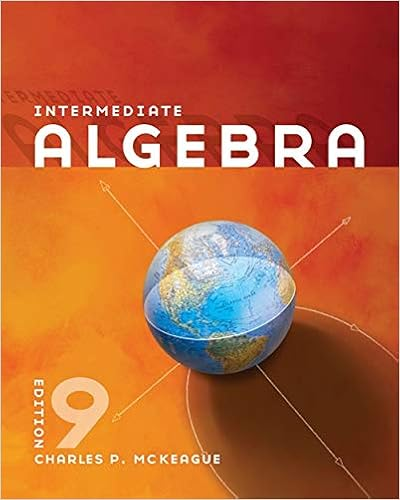# Basically the chain rule states that if changes times

• Test Prep
• 123
• 100% (3) 3 out of 3 people found this document helpful

This preview shows page 91 - 93 out of 123 pages.

##### We have textbook solutions for you!
The document you are viewing contains questions related to this textbook.The document you are viewing contains questions related to this textbook.
Chapter 11 / Exercise 55
Intermediate Algebra
MckeagueExpert Verified
on the right are best done with the Chain Rule. Basically, the Chain Rule states that if changes times as fast as and changes times as fast as then changes times as fast as EXAMPLE 1 The Derivative of a Composite Function A set of gears is constructed, as shown in Figure 2.24, such that the second and third gears are on the same axle. As the first axle revolves, it drives the second axle, which in turn drives the third axle. Let and represent the numbers of revolutions per minute of the first, second, and third axles. Find and and show that Solution Because the circumference of the second gear is three times that of the first, the first axle must make three revolutions to turn the second axle once. Similarly, the second axle must make two revolutions to turn the third axle once, and you can write and Combining these two results, you know that the first axle must make six revolutions to turn the third axle once. So, you can write . In other words, the rate of change of with respect to is the product of the rate of change of with respect to and the rate of change of with respect to x . u u y x y Rate of change of first axle with respect to third axle dy du du dx 3 2 6 Rate of change of second axle with respect to third axle Rate of change of first axle with respect to second axle dy dx du dx 2. dy du 3 dy dx dy du du dx . dy dx , du dx , dy du , x y , u , x . dy du du dx y x , du dx u u , dy du y y x tan x 2 y x tan x y 3 x 2 5 y 3 x 2 y sin 6 x y sin x y x 2 1 y x 2 1 With the Chain Rule Without the Chain Rule 1 1 2 Axle 1 Axle 2 Axle 3 Gear 1 Gear 2 Gear 3 Gear 4 3 Axle 1: revolutions per minute Axle 2: revolutions per minute Axle 3: revolutions per minute Figure 2.24 x u y Video Animation Try It Exploration A
##### We have textbook solutions for you!
The document you are viewing contains questions related to this textbook.The document you are viewing contains questions related to this textbook.
Chapter 11 / Exercise 55
Intermediate Algebra
MckeagueExpert Verified
SECTION 2.4 The Chain Rule 131 Example 1 illustrates a simple case of the Chain Rule. The general rule is stated below. Proof Let Then, using the alternative form of the derivative, you need to show that, for An important consideration in this proof is the behavior of as approaches A problem occurs if there are values of other than such that Appendix A shows how to use the differentiability of and to overcome this problem. For now, assume that for values of other than In the proofs of the Product Rule and the Quotient Rule, the same quantity was added and sub- tracted to obtain the desired form. This proof uses a similar technique—multiplying and dividing by the same (nonzero) quantity. Note that because is differentiable, it is also continuous, and it follows that as When applying the Chain Rule, it is helpful to think of the composite function as having two parts—an inner part and an outer part. Outer function Inner function The derivative of is the derivative of the outer function (at the inner function ) times the derivative of the inner function. y f u u u y f u y f g x f u f g f g c g c lim x c f g x f g c g x g c lim x c g x g c x c lim x c f g x f g c g x g c g x g c x c , g x g c h c lim x c f g x f g c x c x c . g x g c g c . x g x g c g f g x g c . c , x , c . x g h c f g c g c .
•••